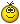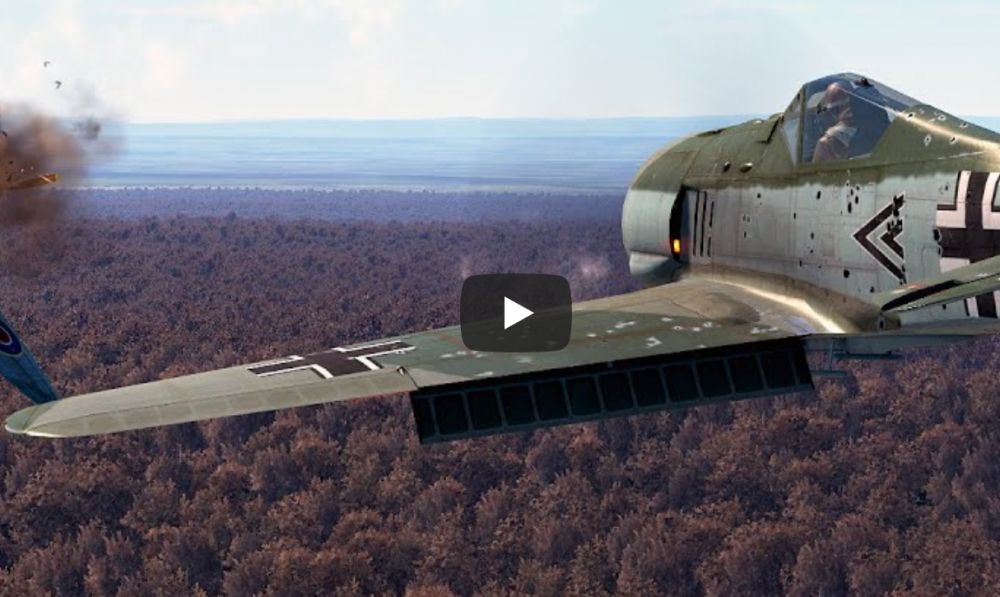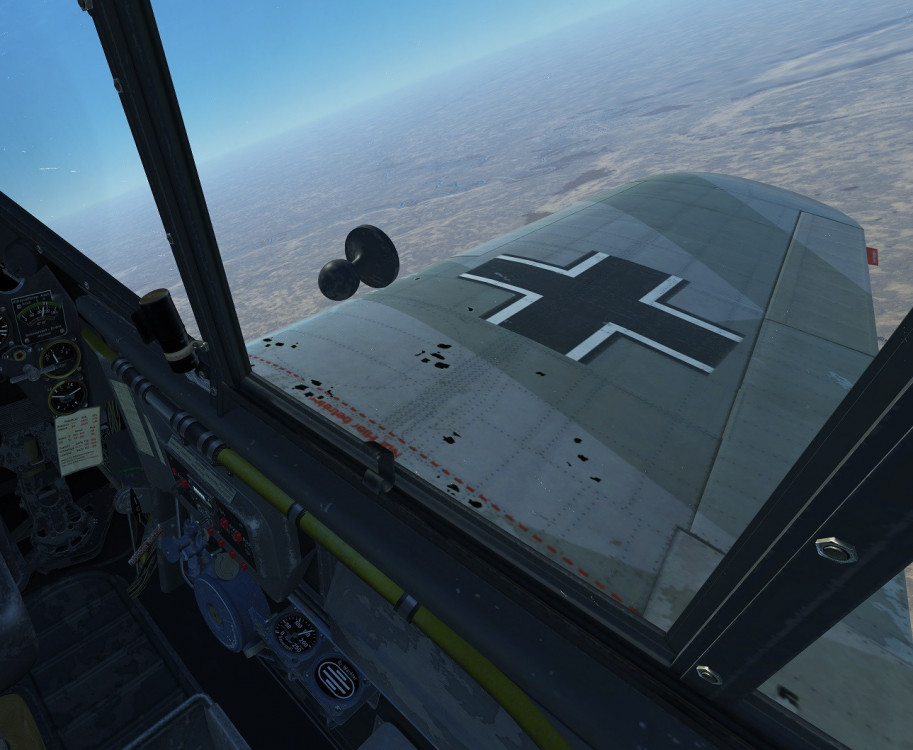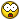# Обсуждение версии 4.001: "Операция Боденплатте" и Flying Circus официально выпущены! Сценарные кампании Tank Crew выпущены!

## Recommended Posts

10 минут назад, BuubGuuL сказал:

вложения средств в то, на чем играешь

никак на влияют на уменьшение вероятности избежать глюков такого рода. В том-то и проблема.

•3
##### Share on other sites
34 минуты назад, AlexVVV сказал:

Уходит в блек без проблем:

...

У меня на фоке даже кнопки на стаб не назначены(надобность отпала после рефератного разжатия рулей)

Есть(сохранился) трек в формате Таквью этого "чудо-самолёта" ?  (посмотрим на Вас/него "глазами цифр")

##### Share on other sites
8 минут назад, -DED-geny сказал:

Есть(сохранился) трек в формате Таквью этого "чудо-самолёта" ?  (посмотрим на Вас/него "глазами цифр")

Которого из?Запись таквью треков ведь отдельной опцией включается?

35 минут назад, Gil--- сказал:А что тандерболт с ними творит - красота##### Share on other sites
3 минуты назад, AlexVVV сказал:

Которого из?Запись таквью треков ведь отдельной опцией включается?

Которые в Вашем "кино" естественно.

Да.

Судя по вопросу -трека нет, а жаль.

##### Share on other sites
58 минут назад, 72AG_Storm сказал:

Полетал на синей стороне на берлоге и какой вывод , у немцев, стаб по умолчанию, ну не реально загнать пилота в блек. Что это заговор?

Вчера бахнулся в лес, слегка круче довернув. Убить пилота противника успел, но и сам - того...

Как у вас не получается блэка выхватить на синих, прям удивляюсь )

К блэку на перегрузках есть и у меня определённые замечания, но я их пока что не совсем четко для себя сформулировал

##### Share on other sites
1 минуту назад, -DED-geny сказал:

Которые в Вашем "кино" естественно.

Да.

Судя по вопросу -трека нет, а жаль.

Я посмотрю. Скорее всего, я выключил эту опцию, т.к., как известно, таквью все врет, сам я РЛЭ не соблюдаю, а место на жестком конечное.

##### Share on other sites
17 минут назад, AlexVVV сказал:

Я посмотрю.

буду признателен.

как известно, таквью все врет

Как известно, цифры пишет БЗХ и надеюсь что они правильные.  Найдем , чем их правильно посмотреть...

##### Share on other sites
1 час назад, AlexVVV сказал:

Уходит в блек без проблем:

У меня на фоке даже кнопки на стаб не назначены(надобность отпала после рефератного разжатия рулей)

УЖАС как лагает, или как модно статерит(((😎

•2
##### Share on other sites
1 час назад, 72AG_Storm сказал:

Полетал на синей стороне на берлоге и какой вывод , у немцев, стаб по умолчанию, ну не реально загнать пилота в блек. Что это заговор? Кто с такими ещё чувствами сталкнулся? Отзавись. То то я все удивлялся раньше ты в блек уходишь , а он летит хоть бы хны и доварачивает до тебя легко , причем скорость у него значительно больше. Становится интересно крутиться, ибо у красных отняли приймущество в ви ражном бою с введением перегрузки. 😉

пробовал его крутить не?

##### Share on other sites
5 минут назад, =LwS=Wize сказал:

пробовал его крутить не?

ПРобовал. Вывод таков . С выкрученным стабом можно уйти в блек , НО это происходит более предсказуемо. На красной стороне очень трудно поймать контроль над блеком. На немцах это проще и легче. На красных ну практически не реально идти в сером)))

Сравни сам. Только не превзято.

Edited by 72AG_Storm
•1
##### Share on other sites
14 минут назад, 72AG_Storm сказал:

ПРобовал. Вывод таков . С выкрученным стабом можно уйти в блек , НО это происходит более предсказуема. На красной стороне очень трудно поймать контроль над блеком. На немцах это проще и легче. На красных ну практически не реально идти в сером)))

привыкли ручку до пупа держать, чего теперь то? Я кстати переучился с новой физикой летать, более плавно теперь ручкой вожу и забросы носом перестал делать ибо бестолку и можно уснуть в неподходящее время, только по прямой и в пологой спирали стараюсь заходить, а красные как уходят резко в сторону вниз так и уходят...так что ничего не поменялось

•5
##### Share on other sites
8 минут назад, 72AG_Storm сказал:

ПРобовал. Вывод таков . С выкрученным стабом можно уйти в блек , НО это происходит более предсказуемо. На красной стороне очень трудно поймать контроль над блеком. На немцах это проще и легче. На красных ну практически не реально идти в сером)))

Сравни сам. Только не превзято.

Теоретически самой жестью в этом отношении должны быть Кобра и Спитфайры. На P-39 в амерском отчете по итогам облета на управляемость и устойчивость отдельно упомянута как недостаток возможность расплющить себя об кресло движением ручки в несколько сантиметров с каким-то смешным усилием.

Edited by Gil---
##### Share on other sites

на красных чувствительность рулей выше, поэтому и в блек легче

15 минут назад, 72AG_Storm сказал:

ПРобовал. Вывод таков . С выкрученным стабом можно уйти в блек , НО это происходит более предсказуемо. На красной стороне очень трудно поймать контроль над блеком. На немцах это проще и легче. На красных ну практически не реально идти в сером)))

Сравни сам. Только не превзято.

пс вернее отзывчивость

Edited by Korch
##### Share on other sites
51 минуту назад, Angriff сказал:

Как у вас не получается блэка выхватить на синих, прям удивляюсь )

Я кстати не помню, говорили об этом что-нибудь или нет, у нас учитываются вещи типа полулежачей позы пилота в фоке? Её собственно ради переносимости перегрузок в реале такой сделали, сколь я помню.

А то может это вот оно и есть.

•1
##### Share on other sites
1 минуту назад, Gil--- сказал:

Я кстати не помню, говорили об этом что-нибудь или нет, у нас учитываются вещи типа полулежачей позы пилота в фоке? Её собственно ради переносимости перегрузок в реале такой сделали, сколь я помню.

А то может это вот оно и есть.

Тут могут ответить только разработчики. Пока ничего не сообщалось про разницу в переносимости, в зависимости от посадки.

##### Share on other sites
2 часа назад, AlexVVV сказал:

Уходит в блек без проблем:

У меня на фоке даже кнопки на стаб не назначены(надобность отпала после рефератного разжатия рулей)

Это, как, .... только не говори что ты так летаешь, это баг записи наверно... Там 10-15 ФПС. Жесть какая.

•1
##### Share on other sites
5 часов назад, Kill_Em_All сказал:

Прошу владельцев карт RTX отписаться, испытывают ли они описанные проблемы с видимостью?

Как у всех смертных. Не только на собственном опыте, но и по разговорам в ТС.

##### Share on other sites

А счётчик 60 показывает😜 где то нас обманывают😜

•1
##### Share on other sites
5 часов назад, KOPCAP сказал:У нас всегда снег!Вам бы из ЛондОнов не знать...

Генератор снега в гусенецах

##### Share on other sites
2 часа назад, 72AGs_miky сказал:

Как у всех смертных. Не только на собственном опыте, но и по разговорам в ТС.

Ну не все смертные недовольны. Кто-то наоборот, пишет что значительно улучшилась ситуация.

Я вот тоже при воспроизведении трека с прошлой версии вижу контакты, которые на старой версии не было видно. Был этому очень удивлен. Да и в самой игре ситуация с видимостью улучшилась после патча по сравнению с тем, что было сразу после введения альтернативной видимости.

Но тут не знаю, с чем это связать - с обновлением игры или с переходом c GTX на RTX, т.к. у меня это было одномоментно...

PS Т.к. трек со старой версии - он воспроизводится - но звук глючит. Не слышно звука оружия и звука двигателя твоего самолета. Окружающие звуки слышно нормально, кроме опять таки оружия.

Edited by Kill_Em_All
##### Share on other sites
‎12‎.‎11‎.‎2019 в 20:33, Slawka сказал:

Хочу попросить прощения у разработчиков и форумчан за своё неправильное поведение на форуме.

Отображение самолёта на сетке прицела сделано достоверно. Я поторопился со своими выводами. Они оказались неверны.

Впредь буду внимательнее.

•6
•3
•1
##### Share on other sites

Уважаемые разработчики, ответьте внятно по проблеме: произвольная раскалибровка осей (любых), выражающаяся в уменьшении ровно в два раза диапазона их действия (сжатии). Проблема старая, писали много раз.

Edited by TUS_Sender
•3
##### Share on other sites
11 часов назад, Kill_Em_All сказал:

Ну не все смертные недовольны. Кто-то наоборот, пишет что значительно улучшилась ситуация.

Я вот тоже при воспроизведении трека с прошлой версии вижу контакты, которые на старой версии не было видно. Был этому очень удивлен. Да и в самой игре ситуация с видимостью улучшилась после патча по сравнению с тем, что было сразу после введения альтернативной видимости.

Я не сравниваю 4.001b с 4.001c, а с видимостью которая была до этого патча. ИМХО она была намного лучше. И это не только исчезновение контактов на близкой дистанции, но и невозможность пользоваться зумом. Там всё перевёрнуто (на определённом расстоянии). При узком FOV контакт намного хуже виден, чем при широком. Так практически невозможно определить дистанцию. На Берлоге можно при вылете увидеть свалку и лететь к ней до бесконечности, пока она не пропадает.

•1
•4
##### Share on other sites
1 час назад, TUS_Sender сказал:

Уважаемые разработчики, ответьте внятно по проблеме: произвольная раскалибровка осей (любых), выражающаяся в уменьшении ровно в два раза диапазона их действия (сжатии). Проблема старая, писали много раз.

Блин, а я думал это у меня джойстик глючит) Так же я думал про сглаживание до того его убрали 😄

Edited by WG_Magners
•2
##### Share on other sites
20 часов назад, AlexVVV сказал:

Уходит в блек без проблем:

У меня на фоке даже кнопки на стаб не назначены(надобность отпала после рефератного разжатия рулей)

Такой лихой заход на спита, как на в самом начале ролика, будь он исполнен на лавке, привел бы к долгому сну пилота с пробуждением в лесу.

•1
•1
•4
##### Share on other sites
56 минут назад, -DED-Hamster сказал:

Такой лихой заход на спита, как на в самом начале ролика, будь он исполнен на лавке, привел бы к долгому сну пилота с пробуждением в лесу.

Как вы это определили, не видя тракетории, показателей скорости, высоты ? Мсье телепат?

##### Share on other sites
10 минут назад, JGr8_Leopard сказал:

Как вы это определили, не видя тракетории, показателей скорости, высоты ? Мсье телепат?

Сеньор! Сеньор телепат!

Edited by -DED-Hamster
##### Share on other sites
2 часа назад, -DED-Hamster сказал:

Такой лихой заход на спита, как на в самом начале ролика, будь он исполнен на лавке, привел бы к долгому сну пилота с пробуждением в лесу.

Садитесь на фоки и все у вас будет хорошо. Но это не точно ©

•2
•1
##### Share on other sites

Когда будет доступна стандартная версия ОБП? И коллекционные самолёты?

•1
##### Share on other sites

После более трёх недель вынужденного простоя, наконец-то вернулся к полетам (офлайн).

Если кратко - восторг!

И как иначе, если:

- картинка идет плавно, идеально (железо в подписи);

- видимость контактов стала значительно лучше;

- появились новые интересные и даже дерзкие нотки в поведении ИИ;

- эффекты перегрузки и накапливания усталости замечательные.

Кстати, что за фантазии, что на синих не словить блэк? Совесть стыда осталась, чтобы такое говорить, по крайней мере про BF-109?

Думаю, синие/полосатые давно привыкли летать аккуратнее из-за зажатых рулей. Поставьте в быстром противников-истребителей "до последнего патрона". После 3-4-го сбитого будете летать в черно-белом кино (усталость накопится), а противники всё свеженькие подваливают.Красные более маневренные, на них тоже пора привыкать летать поаккуратнее. Контроль скорости необходим.

Возможно показалось, что-то новое в ДМ появилось. И разнообразнее, и как-то более достоверно воспринимаемое.

Единственный момент не понял: раньше дырки на крыльях поинтереснее, вроде были, а тут прям кляксы какие-то небрежные. Не было такого и быть не может, сбилось что-то в графике?•1
##### Share on other sites
15.11.2019 в 10:34, Kill_Em_All сказал:

Что-то мнения насчет видимости расходятся... Большенство недовольны, но есть и те, кто наоборот говорит, что все хорошо.

Прошу владельцев карт RTX отписаться, испытывают ли они описанные проблемы с видимостью?

Нужно собрать определенную статистику

Закралась одна мысль тут....

- После недавнего перехода с gtx 1050 ti на RTX 2060 только стабилизировался и вырос ФыПыСы, на видимость контактов никак не повлияло, да и не думаю, что должно повлиять. Как было после обновления почти ничего не видно, так и осталось, как впрочем и у большинства. Печаль ...

Когда уже разработчики займутся видимостью контактов? Большинство вирпилов жалуются, что стало хуже!!!

Что делается в этом направлении?

15.11.2019 в 13:39, AlexVVV сказал:

Уходит в блек без проблем:

У меня на фоке даже кнопки на стаб не назначены(надобность отпала после рефератного разжатия рулей)

Как можно летать с такими статтерами??? Жуть! Глаза не отваливаются??

•1
##### Share on other sites
16 минут назад, SkvK_Tico-Tico сказал:

Как можно летать с такими статтерами???

Раз в месяц.

##### Share on other sites
1 час назад, AlexVVV сказал:

Раз в месяц.

А потом к окулисту на месяц?)))

Не бережете вы себя...

•1
##### Share on other sites
15.11.2019 в 15:39, AlexVVV сказал:

после рефератного разжатия рулей

честно гуглил и яндыксил,но так и не понял,что это значит##### Share on other sites
9 часов назад, -DED-Hamster сказал:

Такой лихой заход на спита, как на в самом начале ролика, будь он исполнен на лавке, привел бы к долгому сну пилота с пробуждением в лесу.

Я вот считаю наоборот, такие уходы из под атаки у темпестов должны сопровождаться глубоким блэком....)))) И я крайне удивлен, почему темпесты не ловили БЛЭКК!!!1111

Прошу разработчиков обратить на это ОСОБОЕ ВНИМАНИЕ

))))

видишь, сколько людей столько мнений....

То рассудит нас либо трек, либо запись, либо ТакВью (которая не факт, что правильно рассудит) ))))))

##### Share on other sites
14 минут назад, Kill_Em_All сказал:

Я вот считаю наоборот, такие уходы из под атаки у темпестов должны сопровождаться глубоким блэком....)))) И я крайне удивлен, почему темпесты не ловили БЛЭКК!!!1111

Там скорость нулевая.

##### Share on other sites
15 минут назад, 72AGs_miky сказал:

Там скорость нулевая.

))))))))))))))))))))))))))))))))))))))))))))))))))))))))))))))))))))))))))))))))))))))))))))))))))))))))))))))))))))))))

))))))))))))))))))))))))))))))))))))))))))))))))))))))))))))))))))))))))))))))))))))))))))))))))))))))))))))))))))))))))

))))))))))))))))))))))))))))))))))))))))))))))))))))))))))))))))))))))))))))))))))))))))))))))))))))))))))))))))))))))))

))))))))))))))))))))))))))))))))))))))))))))))))))))))))))))))))))))))))))))))))))))))))))))))))))))))))))))))))))))))))

##### Share on other sites
43 минуты назад, Kill_Em_All сказал:

Я вот считаю наоборот, такие уходы из под атаки у темпестов должны сопровождаться глубоким блэком....)))) И я крайне удивлен, почему темпесты не ловили БЛЭКК!!!1111

Прошу разработчиков обратить на это ОСОБОЕ ВНИМАНИЕ

))))

видишь, сколько людей столько мнений....

То рассудит нас либо трек, либо запись, либо ТакВью (которая не факт, что правильно рассудит) ))))))

Да ловятся блэки на темпестах, только в путь. С "коротким" джоем очень неудобно контролировать перегрузку, постоянно улетаешь в несознанку. Может с подстульниками-напольниками парням проще..

##### Share on other sites
38 минут назад, Red_Pilot сказал:

Да ловятся блэки на темпестах, только в путь.С "коротким" джоем очень неудобно контролировать перегрузку, постоянно улетаешь в несознанку. Может с подстульниками-напольниками парням проще..

Да ловятся блэки на ФОКАХ, только в путь.С "коротким" джоем очень неудобно контролировать перегрузку, постоянно улетаешь в несознанку. Может с подстульниками-напольниками парням проще..

Эта фраза принеминима к ЛЮБЫм самолетам в игре.

Вопрос лишь в том, на темной или на светлой стороне силы ты. Интересы той стороны отстаивать и будешь...

•1
•3
##### Share on other sites
51 минуту назад, Red_Pilot сказал:

Да ловятся блэки на ХХХ, только в путь. С "коротким" джоем очень неудобно контролировать перегрузку, постоянно улетаешь в несознанку. Может с подстульниками-напольниками парням проще..

Вместо ХХХ - любой самолет с небольшим запасом устойчивости. Потому ручкой нужной двигать в небольших пределах. На длинноходных ручках это соответственно проще.

Edited by JGr8_Leopard
•1
##### Share on other sites
• Han locked and unlocked this topic
• Han unpinned this topic